Next: Entropy Production Up: Plasma Fluid Theory Previous: Moments of the Kinetic

# Fluid Equations

It is conventional to rewrite our fluid equations in terms of the pressure tensor,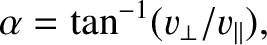, and the heat flux density,. Substituting from Eqs. (190)-(191), and performing a little tensor algebra, Eqs. (209)-(211) reduce to: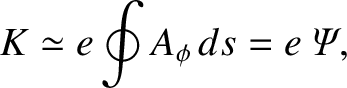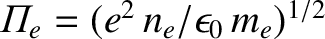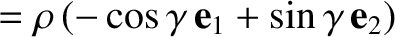(214)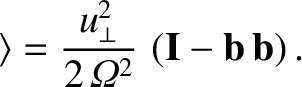(215)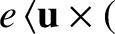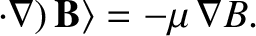(216)

Here,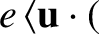(217)

is the well-known convective derivative, and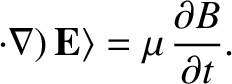(218)

In the above,and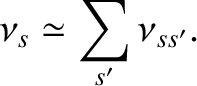refer to Cartesian components, and repeated indices are summed (according to the Einstein summation convention). The convective derivative, of course, measures time variation in the local rest frame of the species-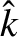fluid. Strictly speaking, we should include ansubscript with each convective derivative, since this operator is clearly different for different plasma species.

There is one additional refinement to our fluid equations which is worth carrying out. We introduce the generalized viscosity tensor,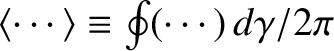, by writing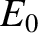(219)

where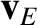is the unit (identity) tensor. We expect the scalar pressure term to dominate if the plasma is relatively close to thermal equilibrium. We also expect, by analogy with conventional fluid theory, the second term to describe viscous stresses. Indeed, this is generally the case in plasmas, although the generalized viscosity tensor can also include terms which are quite unrelated to conventional viscosity. Equations (214)-(216) can, thus, be rewritten:(220)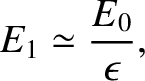(221)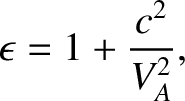(222)

According to Eq. (220), the species-density is constant along a fluid trajectory unless the species-flow is non-solenoidal. For this reason, the condition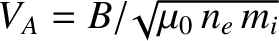(223)

is said to describe incompressible species-flow. According to Eq. (221), the species-flow accelerates along a fluid trajectory under the influence of the scalar pressure gradient, the viscous stresses, the Lorentz force, and the frictional force due to collisions with other species. Finally, according to Eq. (222), the species-energy density (i.e.,) changes along a fluid trajectory because of the work done in compressing the fluid, viscous heating, heat flow, and the local energy gain due to collisions with other species. Note that the electrical contribution to plasma heating, which was explicit in Eq. (211), has now become entirely implicit.Next: Entropy Production Up: Plasma Fluid Theory Previous: Moments of the Kinetic
Richard Fitzpatrick 2011-03-31